MARGINAL UTILITY In relation to consumers MARGINAL UTILITY

• Slides: 22MARGINAL UTILITY In relation to consumers!!!!!!MARGINAL UTILITY • Marginal = Extra • Utility = Satisfaction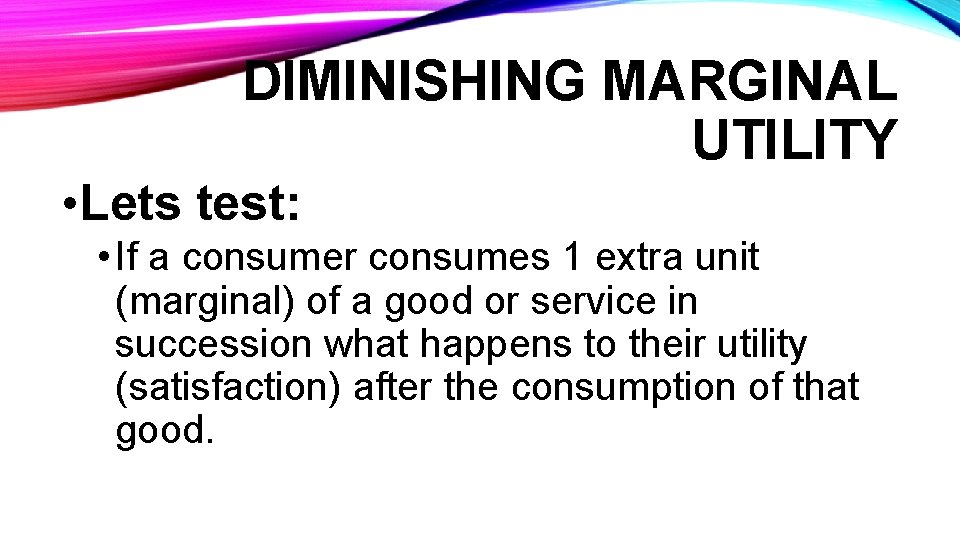DIMINISHING MARGINAL UTILITY • Lets test: • If a consumer consumes 1 extra unit (marginal) of a good or service in succession what happens to their utility (satisfaction) after the consumption of that good.Yum 10 out of 10 Ok 8 out of 10 Ghosty 6 out of 10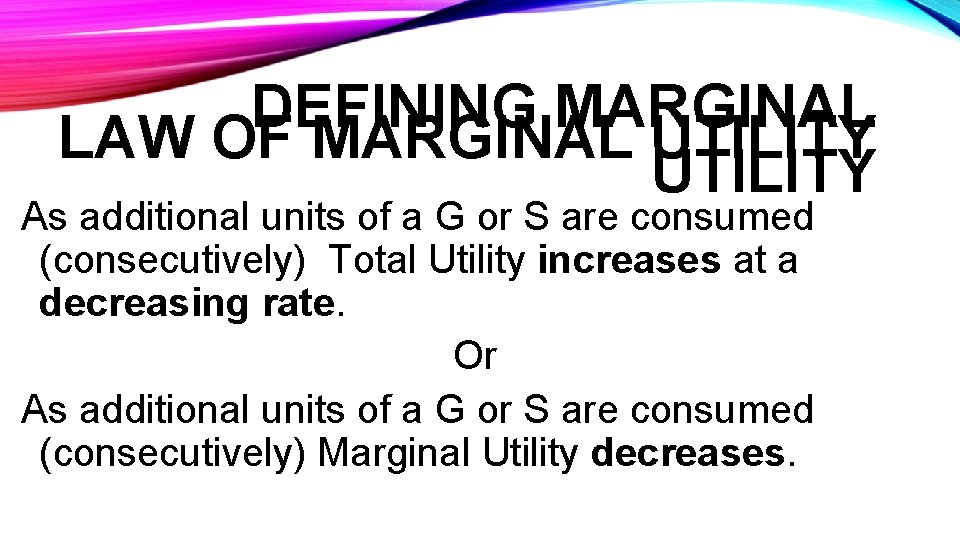DEFINING MARGINAL LAW OF MARGINAL UTILITY As additional units of a G or S are consumed (consecutively) Total Utility increases at a decreasing rate. Or As additional units of a G or S are consumed (consecutively) Marginal Utility decreases.CRITERIA • LI: Understand apply the Law of Diminishing Marginal UtilityREVISIT Yum 10 out of 10 Ok 8 out of 10 Ghosty 6 out of 10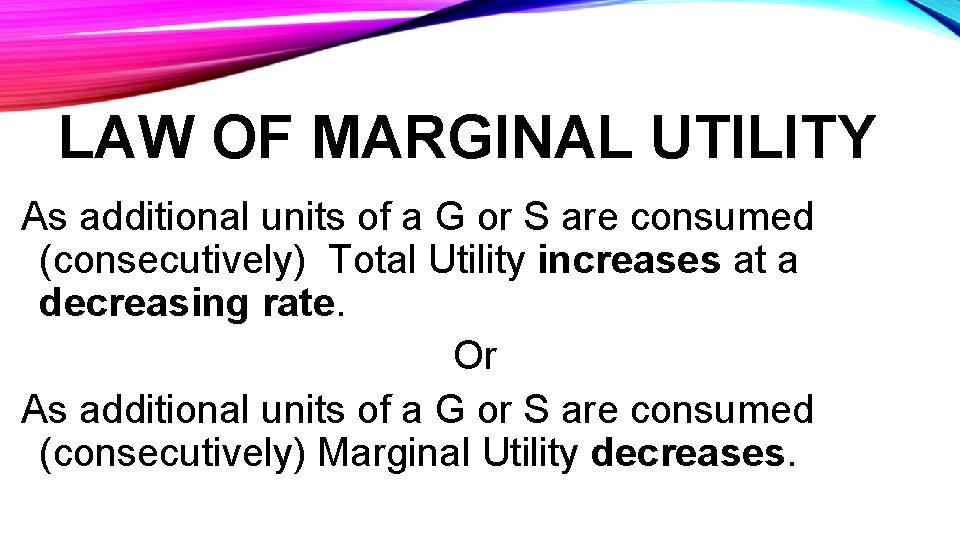LAW OF MARGINAL UTILITY As additional units of a G or S are consumed (consecutively) Total Utility increases at a decreasing rate. Or As additional units of a G or S are consumed (consecutively) Marginal Utility decreases.THE OPTIMUM PURCHASE RULE P = MU • Consumers will/should continue to consume up to where Price is equal to Marginal Utility (P = MU) as they will maximise their total utility.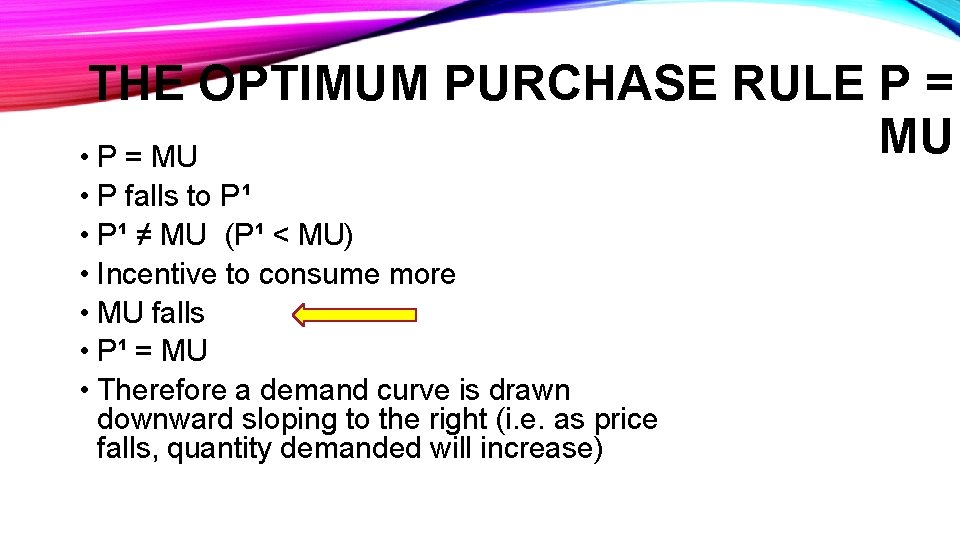THE OPTIMUM PURCHASE RULE P = MU • P falls to P¹ • P¹ ≠ MU (P¹ < MU) • Incentive to consume more • MU falls • P¹ = MU • Therefore a demand curve is drawn downward sloping to the right (i. e. as price falls, quantity demanded will increase)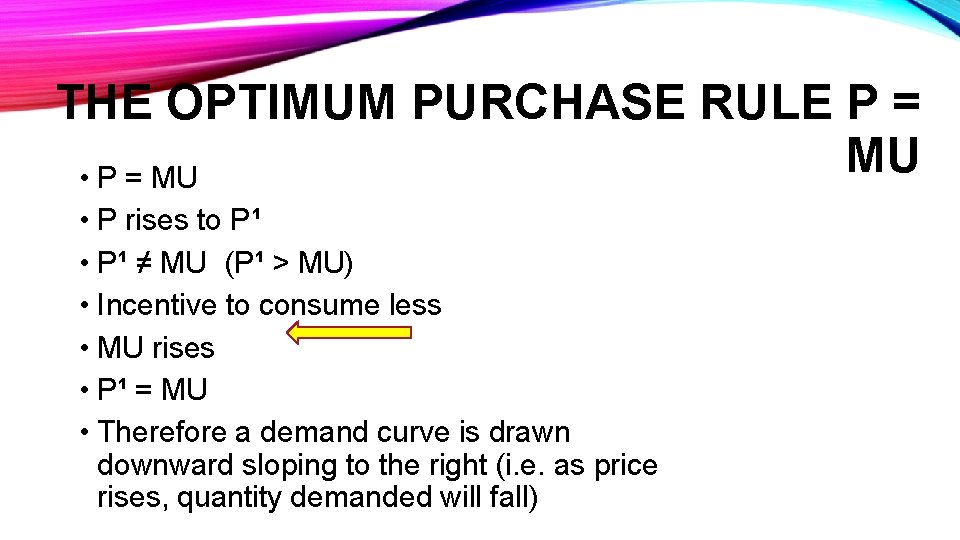THE OPTIMUM PURCHASE RULE P = MU • P rises to P¹ • P¹ ≠ MU (P¹ > MU) • Incentive to consume less • MU rises • P¹ = MU • Therefore a demand curve is drawn downward sloping to the right (i. e. as price rises, quantity demanded will fall)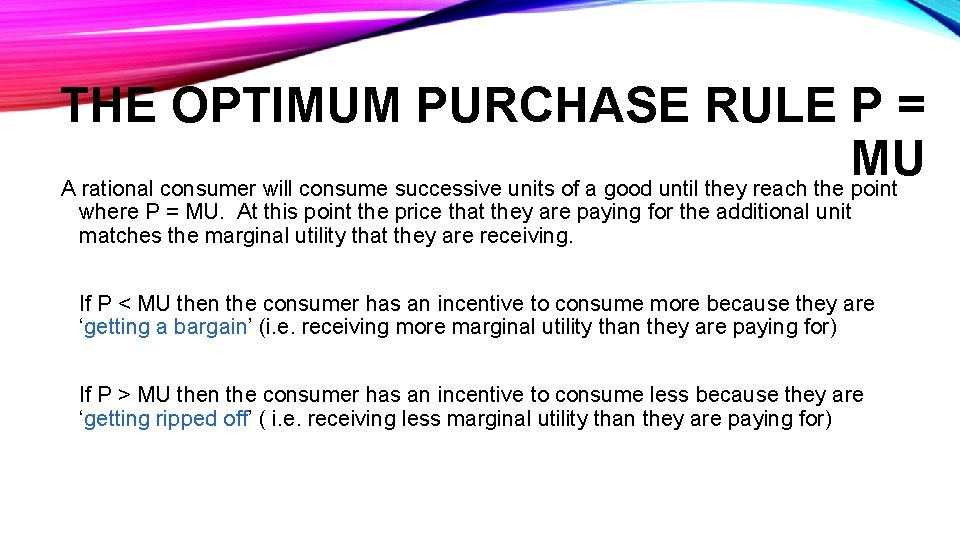THE OPTIMUM PURCHASE RULE P = MU A rational consumer will consume successive units of a good until they reach the point where P = MU. At this point the price that they are paying for the additional unit matches the marginal utility that they are receiving. If P < MU then the consumer has an incentive to consume more because they are ‘getting a bargain’ (i. e. receiving more marginal utility than they are paying for) If P > MU then the consumer has an incentive to consume less because they are ‘getting ripped off’ ( i. e. receiving less marginal utility than they are paying for)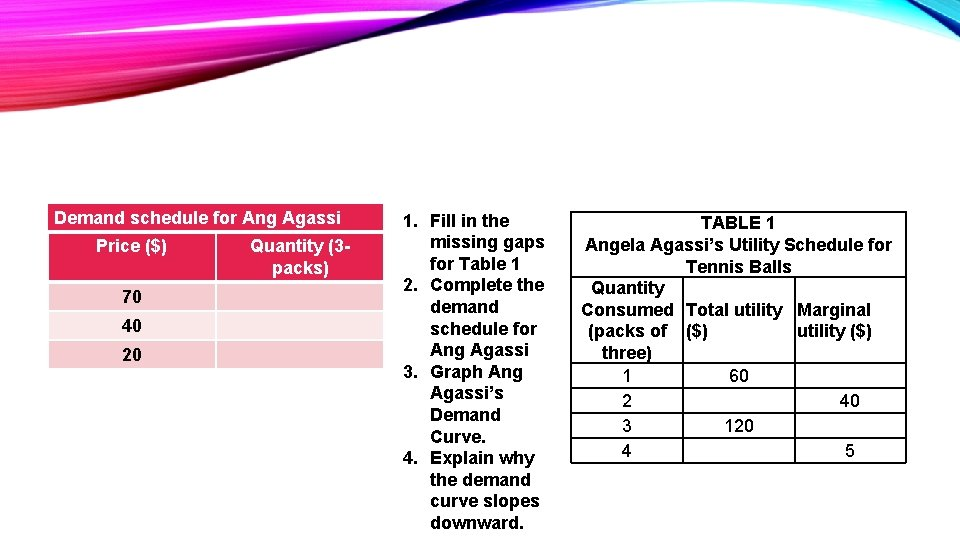Demand schedule for Ang Agassi Price (\$) 70 40 20 Quantity (3 packs) 1. Fill in the missing gaps for Table 1 2. Complete the demand schedule for Ang Agassi 3. Graph Ang Agassi’s Demand Curve. 4. Explain why the demand curve slopes downward. TABLE 1 Angela Agassi’s Utility Schedule for Tennis Balls Quantity Consumed Total utility Marginal (packs of (\$) utility (\$) three) 1 60 2 40 3 120 4 5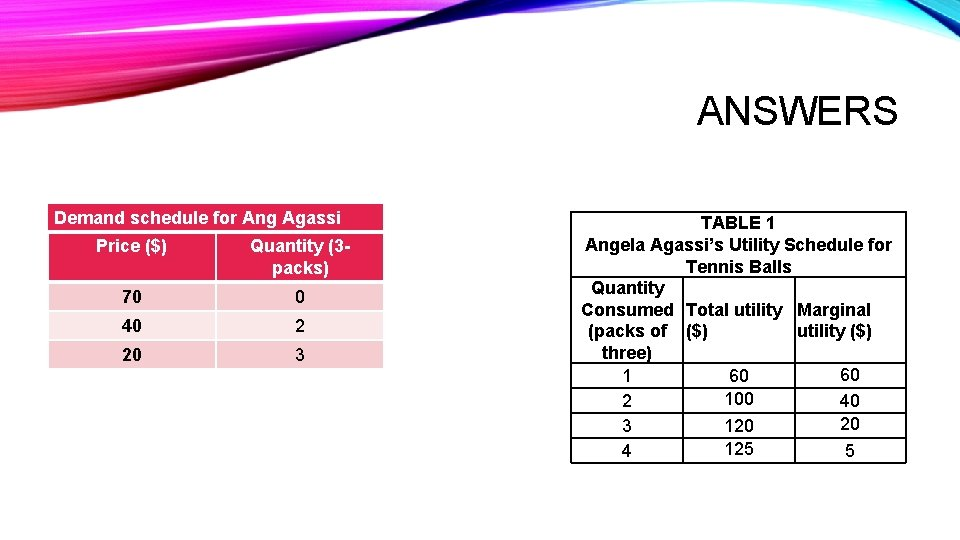ANSWERS Demand schedule for Ang Agassi Price (\$) Quantity (3 packs) 70 0 40 2 20 3 TABLE 1 Angela Agassi’s Utility Schedule for Tennis Balls Quantity Consumed Total utility Marginal (packs of (\$) utility (\$) three) 60 100 2 40 20 3 120 125 4 5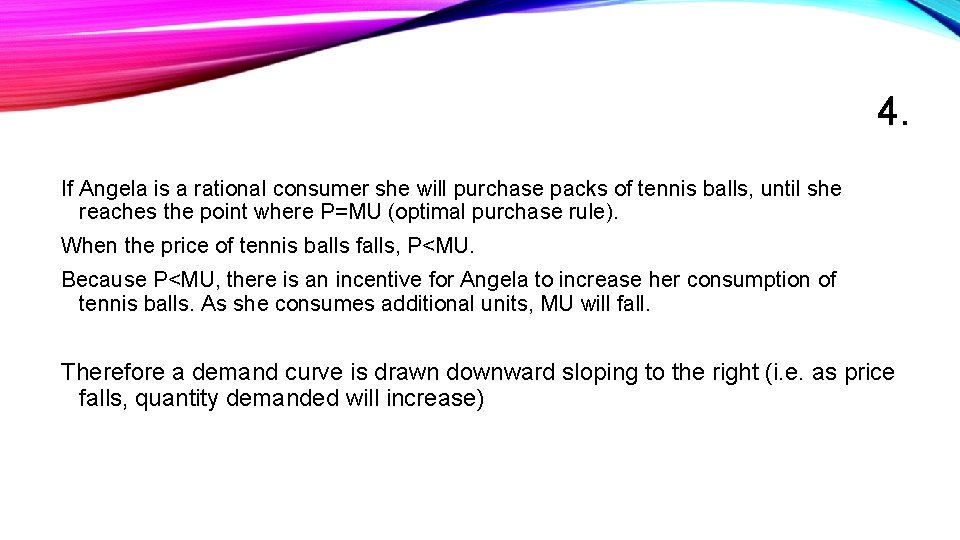4. If Angela is a rational consumer she will purchase packs of tennis balls, until she reaches the point where P=MU (optimal purchase rule). When the price of tennis balls falls, P<MU. Because P<MU, there is an incentive for Angela to increase her consumption of tennis balls. As she consumes additional units, MU will fall. Therefore a demand curve is drawn downward sloping to the right (i. e. as price falls, quantity demanded will increase)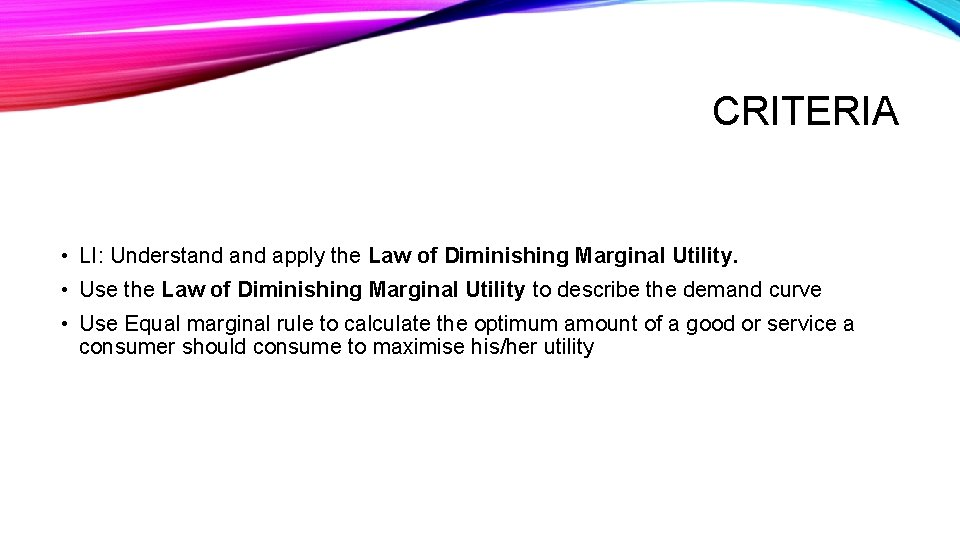CRITERIA • LI: Understand apply the Law of Diminishing Marginal Utility. • Use the Law of Diminishing Marginal Utility to describe the demand curve • Use Equal marginal rule to calculate the optimum amount of a good or service a consumer should consume to maximise his/her utility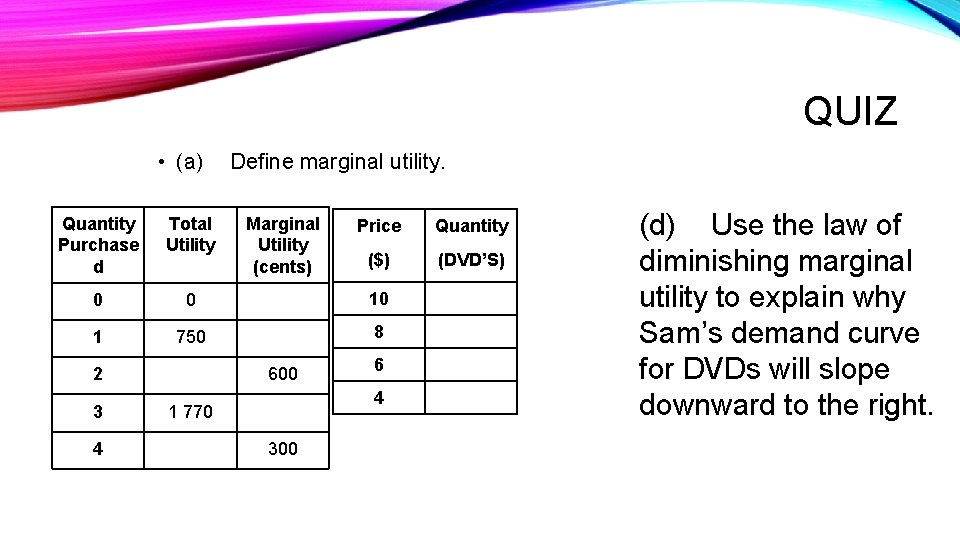QUIZ • (a) Define marginal utility. Quantity Purchase d Total Utility 0 0 10 1 750 8 2 3 4 Marginal Utility (cents) 600 Price Quantity (\$) (DVD’S) 6 4 1 770 300 (d) Use the law of diminishing marginal utility to explain why Sam’s demand curve for DVDs will slope downward to the right.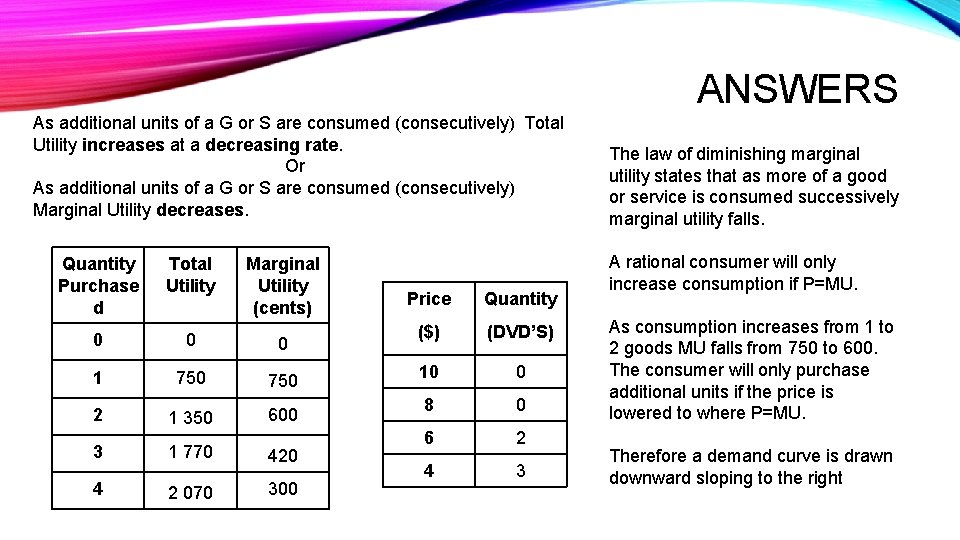ANSWERS As additional units of a G or S are consumed (consecutively) Total Utility increases at a decreasing rate. Or As additional units of a G or S are consumed (consecutively) Marginal Utility decreases. Quantity Purchase d Total Utility 0 0 0 1 750 1 350 600 3 1 770 420 4 2 070 300 2 Marginal Utility (cents) Price Quantity (\$) (DVD’S) 10 0 8 0 6 2 4 3 The law of diminishing marginal utility states that as more of a good or service is consumed successively marginal utility falls. A rational consumer will only increase consumption if P=MU. As consumption increases from 1 to 2 goods MU falls from 750 to 600. The consumer will only purchase additional units if the price is lowered to where P=MU. Therefore a demand curve is drawn downward sloping to the right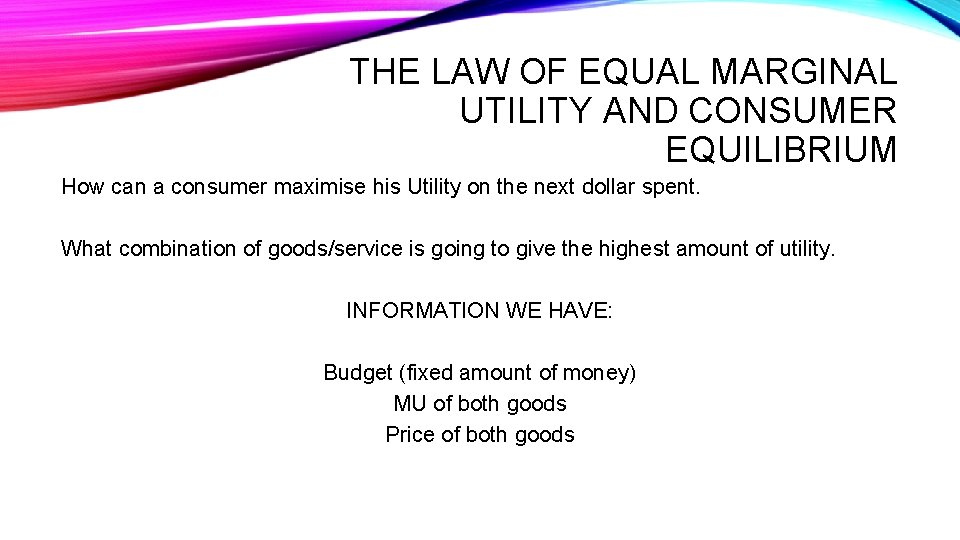THE LAW OF EQUAL MARGINAL UTILITY AND CONSUMER EQUILIBRIUM How can a consumer maximise his Utility on the next dollar spent. What combination of goods/service is going to give the highest amount of utility. INFORMATION WE HAVE: Budget (fixed amount of money) MU of both goods Price of both goods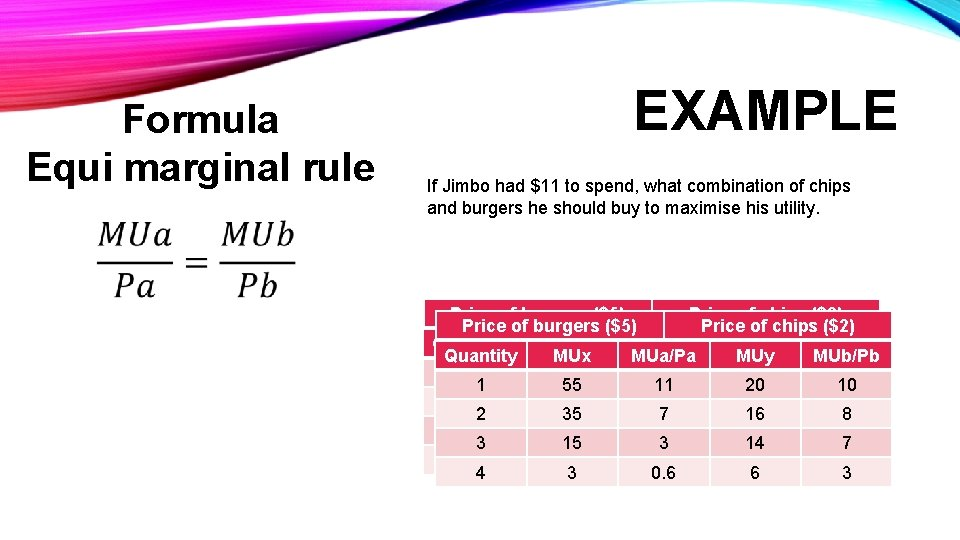Formula Equi marginal rule EXAMPLE If Jimbo had \$11 to spend, what combination of chips and burgers he should buy to maximise his utility. Price of burgers (\$5) Price of chips (\$2) Quantity MUx MUa/Pa MUy MUb/Pb 1 55 20 1 55 11 20 10 2 35 16 2 35 7 16 8 3 15 14 3 15 3 14 7 4 3 6 4 3 0. 6 6 3QUICK QUIZ • Jack and Jill both earn \$10 pocket money. They spend all \$10 on chips and lollies. Jill purchases six packets of chips and two packets of lollies. • Use table 1 to determine the quantity of chips and lollies Jack should purchase to maximise his total utility. • A packet of chips costs \$1 and a packet of lollies costs \$2. Quantity of Marginal utility MUa/MUb chips (packet) (utils) chips lollies (packet) (utils) lollies 1 400 2 350 2 300 3 250 3 200 4 100 5 50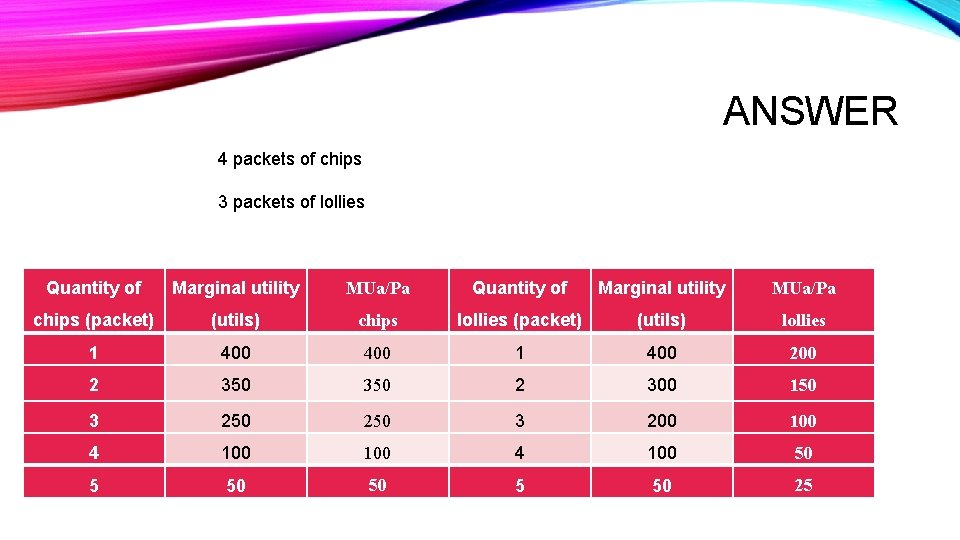ANSWER 4 packets of chips 3 packets of lollies Quantity of Marginal utility MUa/Pa chips (packet) (utils) chips lollies (packet) (utils) lollies 1 400 2 350 2 300 150 3 250 3 200 100 4 100 50 5 50 25